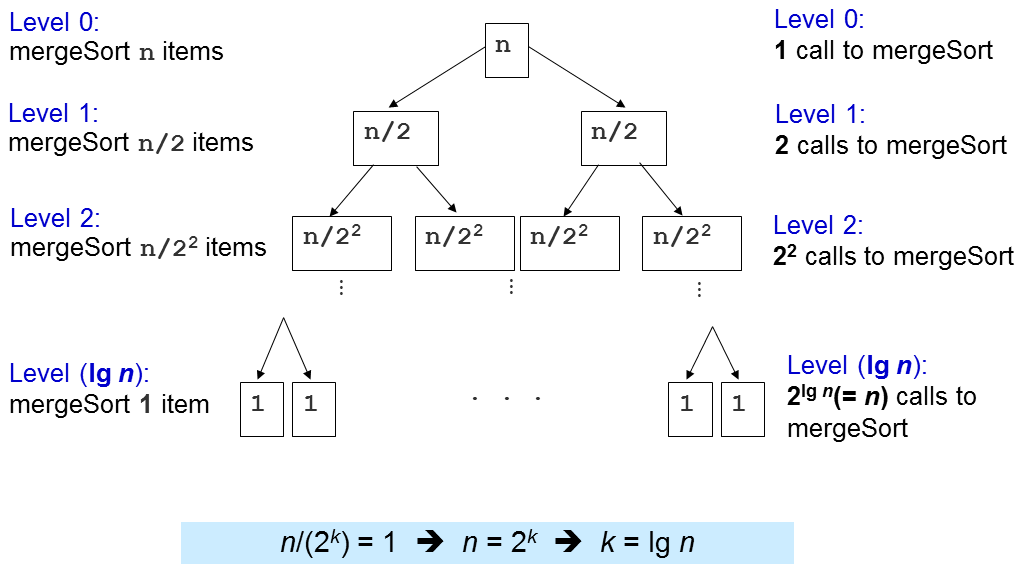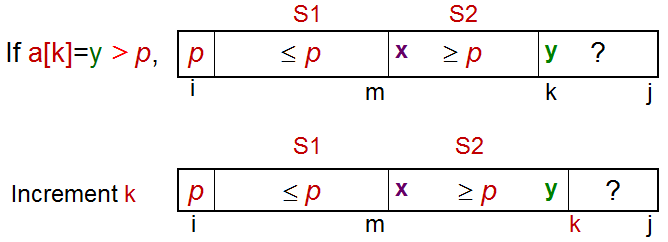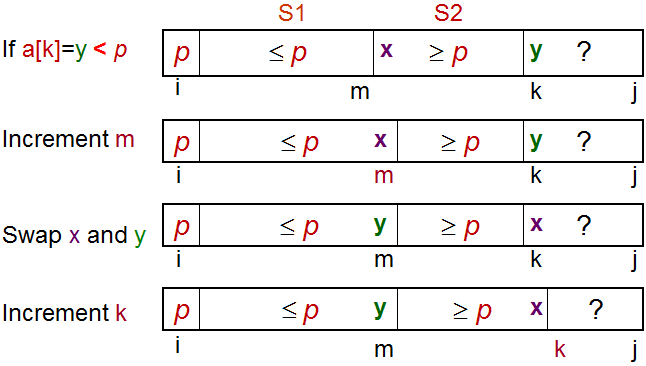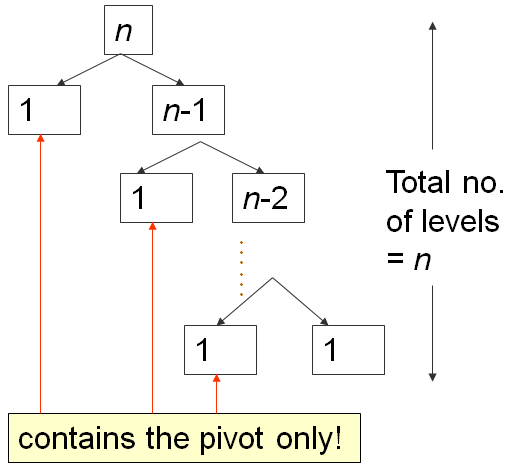# 排序问题和排序算法

## 1. Introduction

### 1-1. 动力 - 有趣的计算机科学想法

Sorting problem has a variety of interesting algorithmic solutions that embody many Computer Science ideas:

1. Comparison versus non-comparison based strategies,
2. Iterative versus Recursive implementation,
3. Divide-and-Conquer paradigm (e.g., Merge Sort or Quick Sort),
4. Best/Worst/Average-case Time Complexity analysis,
5. Randomized Algorithms, etc.

### 1-2. 动力 - 应用

1. 在数组 A 中搜索特定值 v
2. 查找（静态）数组 A 中的最小/最大/第 k 个最小/最大值，
3. 测试唯一性并删除数组 A 中的重复项，
4. 计算特定值 v 在数组 A 中出现多少次，
5. 设置数组 A 和另一个排序数组 B 之间的交集/联合，
6. 寻找一个目标对 x∈Ay∈A，使得 x + y 等于目标 z 等。
7. 计算在区间 [lo..hi] 内共计有多少个值。

### 1-3. 小提示

[This is a hidden slide]

## 2. 动作

### 2-1. 定义你自己的输入

1. 完全随机的
2. 随机但有序的（按升序/降序排列）
3. 随机但几乎有序的（按升序/降序排列）
4. 随机但包含许多重复项（因此在小范围的整数内）
5. 完全由你自己定义。

## 4. 常见的排序算法

### 4-1. 缩写

1. 基于比较的排序算法:
1. BUB - 冒泡排序，
2. SEL - 选择排序，
3. INS - 插入排序，
4. MER - 归并排序 (递归实现)，
5. QUI - 快速排序 (递归实现)，
6. R-Q - 随机快速排序 (递归实现)
2. 不基于比较的排序算法:
1. COU - 计数排序，

## 6. 基础算法分析

### 6-1. 数学预备知识

-. 对数和指数, e.g., log2(1024) = 10, 210 = 1024
-. 等差数列, e.g., 1+2+3+4+…+10 = 10*11/2 = 55
-. 等比数列, e.g., 1+2+4+8+..+1024 = 1*(1-211)/(1-2) = 2047
-. 线性/二次/三次函数, e.g., f1(x) = x+2, f2(x) = x2+x-1, f3(x) = x3+2x2-x+7
-. 向上取整 (ceiling), 向下取整 (floor) , 和绝对值 (absolute) 函数, e.g., ceil(3.1) = 4, floor(3.1) = 3, abs(-7) = 7

### 6-6. 渐进分析

f(1000) = 2*10002 + 100*1000 = 2.1M, vs
f(100000) = 2*1000002 + 100*100000 = 20010M。

### 6-10. 增长项

O(1)/常数时间 < O(log n)/对数时间 < O(n)/线性时间 < O(n log n)/准线性时间 < O(n2)/二次时间 < O(n3)/三次时间 <
O(2n)/指数时间 < O(n!)/同样指数时间 < ∞ (如死循环).

## 7. 冒泡排序

1. 比较一对相邻元素（a，b），
2. 如果两项的大小关系不正确，交换这两个数（在本例中为a > b），
3. 重复步骤1和2，直到我们到达数组的末尾（最后一对是第 N-2 N-1 项，因为我们的数组从零开始）
4. 到目前为止，最大项将在最后的位置。 然后我们将 N 减少1，并重复步骤1，直到 N = 1

### 7-1. 冒泡排序：C++代码及分析

`void bubbleSort(int a[], int N) { // 标准版本  for (; N > 0; --N) // N次迭代    for (int i = 0; i < N-1; ++i) // 除最后一次, O(N)      if (a[i] > a[i+1]) // 若数组元素i和i+1成非递减次序        swap(a[i], a[i+1]); // 在O(1)时间内交换}`

（标准）冒泡排序中有两个嵌套循环。

1. 当 i = 0，内循环进行N-1次比较和交换的迭代；
2. 当 i = 1，N-2次迭代；
3. 当 i = N-2 时，1次迭代；
4. 当 i = N-1 时，0次迭代。

### 7-3. 答案

[This is a hidden slide]

## 8. 选择排序

1. [L ... N-1] 范围内找出最小项目 X 的位置，
2. 用第 L 项交换第 X 项，
3. 将下限 L 增加1并重复步骤1直到 L = N-2

### 8-1. 选择排序，C++代码及分析

`void selectionSort(int a[], int N) {  for (int L = 0; L <= N-2; L++) { // O(N)    找到[L,N-1]区间的最小的数的序号X // O(N)    swap(X, L) // O(1), 注意X可能等于L（没有交换）  }}`

### 8-2. 小测验

Quiz: How many (real) swaps are required to sort [29, 10, 14, 37, 13] by Selection Sort?

3
4
2
1

## 9. 插入排序

1. 从你手中的一张牌开始，
2. 选择下一张牌并将其插入到正确的排序顺序中，
3. 对所有牌重复上一步。

### 9-1. 插入排序，C++ 代码及分析 1

`void insertionSort(int a[], int N) { //插入排序  for (int i = 1; i < N; i++) { // O(N)    X = a[i]; // X 是要被插入的那个数    for (j = i-1; j >= 0 && a[j] > X; j--) // 速度有快有慢      a[j+1] = a[j]; // 为 X 腾出一个空间    a[j+1] = X; // 此处为插入点  }}`

### 9-2. 插入排序：分析 2

1. 在最好的情况下，数组已经排序并且 (a [j]> X) 总是为假。所以不需要移位数据，并且内部循环在O（1）时间内运行，
2. 在最坏的情况下，数组被反向排序并且 (a [j]> X) 总是为真。插入始终发生在数组的前端，并且内部循环在O（N）时间内运行。

### 9-3. 小测验

Quiz: What is the complexity of Insertion Sort on any input array?

O(N log N)
O(1)
O(N)
O(N^2)

## 10. 2.5 O(N log N) 基于比较的排序

1. 归并排序
2. 快速排序和它的随机版本（只有一个变化）。

PS：尽管如此，非随机版本的快速排序（Quick Sort）需要 O(N2) 时间来运行

## 11. 归并排序

1. 将每对单个元素（默认情况下，已排序）归并为2个元素的有序数组，
2. 将2个元素的每对有序数组归并成4个元素的有序数组，重复这个过程......，
3. 最后一步：归并2个N / 2元素的排序数组（为了简化讨论，我们假设N是偶数）以获得完全排序的N个元素数组。

### 11-2. 归并子程序 C++ 实现方法

`void merge(int a[], int low, int mid, int high) {  // subarray1 = a[low..mid], subarray2 = a[mid+1..high], both sorted  int N = high-low+1;  int b[N]; // 讨论: 为什么我们需要一个临时的数组 b?  int left = low, right = mid+1, bIdx = 0;  while (left <= mid && right <= high) // 归并    b[bIdx++] = (a[left] <= a[right]) ? a[left++] : a[right++];  while (left <= mid) b[bIdx++] = a[left++]; // leftover, if any  while (right <= high) b[bIdx++] = a[right++]; // leftover, if any  for (int k = 0; k < N; k++) a[low+k] = b[k]; // copy back}`

### 11-3. 分而治之的范例

1. 划分步骤：将大的原始问题划分成较小的子问题并递归地解决较小的子问题，
2. 解决步骤：结合较小的子问题的结果来解决较大的原始问题。

### 11-5. 归并排序的 C++ 实现方法

`void mergeSort(int a[], int low, int high) {  // 要排序的数组是 a[low..high]  if (low < high) { // 基础情况: low >= high (0或1项)    int mid = (low+high) / 2;	    mergeSort(a, low  , mid ); // 分成两半    mergeSort(a, mid+1, high); // 递归地将它们排序    merge(a, low, mid, high); // 解决步骤: 归并子程序  }}`

### 11-8. 归并排序：分析 第二部分...

### 11-11. 答案

[This is a hidden slide]

## 12. 快速排序

### 12-2. 重要的子程序，O(N) 分区

1. S1 = a [i + 1..m]其中项目 ≤ p
2. S2 = a [m + 1..k-1]，其中项目 ≥ p，且
3. 未知的= a [k..j]，其中项目尚未分配给 S1 S2

### 12-3. 答案

[This is a hidden slide]

### 12-4. 分区排序 - 继续

1. 如果 a[k] > p，将 a[k] 放入 S2
2. 如果 a[k] < p，将 a[k] 放入 S1
3. 如果 a[k] == p，扔硬币决定将 a[k] 放入 S2 还是 S1 中。

### 12-5. 分区 - 当 a[k] > p 的情况### 12-6. 分区 - 当  a[k] < p 的情况### 12-7. 分区 C++ 实现方法

`int partition(int a[], int i, int j) {  int p = a[i]; // p 是 pivot  int m = i; // S1 和 S2 一开始是空的  for (int k = i+1; k <= j; k++) { // 探索未知的区域    if (a[k] < p) { // case 2      m++;      swap(a[k], a[m]); // C++ STL algorithm std::swap    } // 注意：在情况1的时候我们什么都不做: a[k] >= p  }  swap(a[i], a[m]); // 最后一步, 用a[m]交换 pivot  return m; // 返回pivot的索引, 用于快速排序（Quick Sort）}`

### 12-8. 快速排序 C++ 实现方法

`void quickSort(int a[], int low, int high) {  if (low < high) {    int m = partition(a, low, high); // O(N)    // a[low..high] ~> a[low..m–1], pivot, a[m+1..high]    quickSort(a, low, m-1); // 递归地将左侧子数列排序    // a[m] = pivot 在分区后就被排序好了    quickSort(a, m+1, high); // 然后将右侧子数列排序  }}`

### 12-12. 快速排序： 分析 第三部分第一次分区需要 O(N) 时间，将 a 分成 0, 1, N-1个项，然后向右递归。

...

## 13. 随机快速排序

### 13-2. 答案

[This is a hidden slide]

1. 计数排序,
2. 基数排序.

## 16. 基数排序

### 16-2. 答案

[This is a hidden slide]

## 17. 排序算法的附加属性

### 17-3. 缓存性能

[This is a hidden slide]

## 18. 小测验

### 18-1. 小测验 #1

Quiz: Which of these algorithms run in O(N log N) on any input array of size N?

Insertion Sort
Merge Sort
Quick Sort (Deterministic)
Bubble Sort

### 18-2. 小测验 #2

Quiz: Which of these algorithms has worst case time complexity of Θ(N^2) for sorting N integers?

Bubble Sort
Merge Sort
Selection Sort